Register for Maths, Science, English, Reasoning Olympiad Exams Register here | Book Free Demo for Live Olympiad Classes here | Check Olympiad Exam Dates here | Buy Practice Papers for IMO, IOM, HEO, IOEL etc here | Login here to participate in all India free mock test on every Saturday

# Point, Line and Plane Figures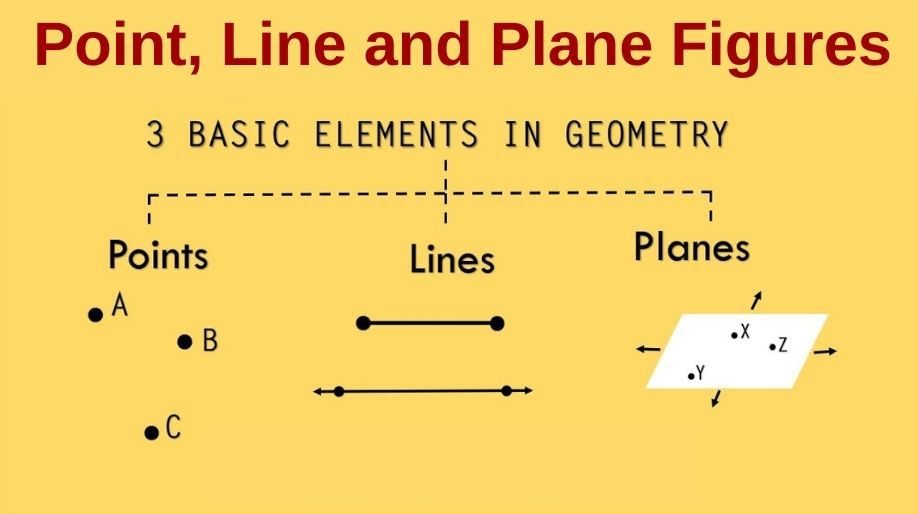What is a point? Point: A point is a dot or other punctuation mark, in particular, a full stop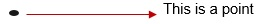Line:
a) It is straight.
b) Has no thickness.
c) Extends in both directions.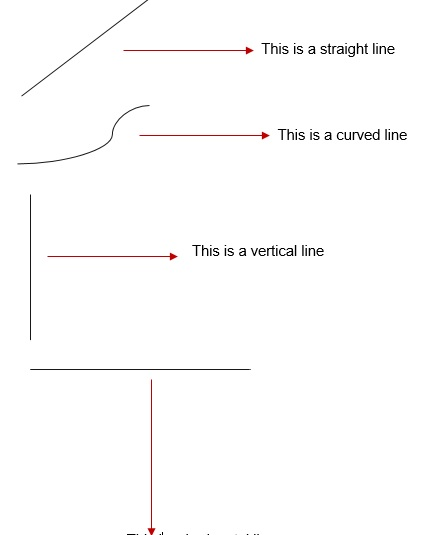This is a horizontal line

# Plane Figures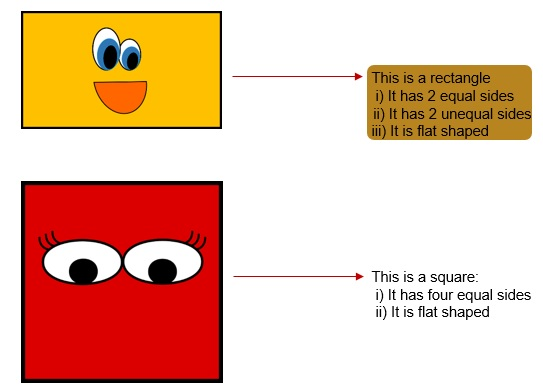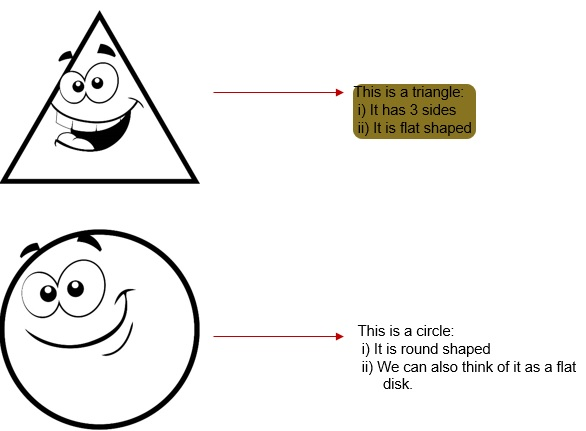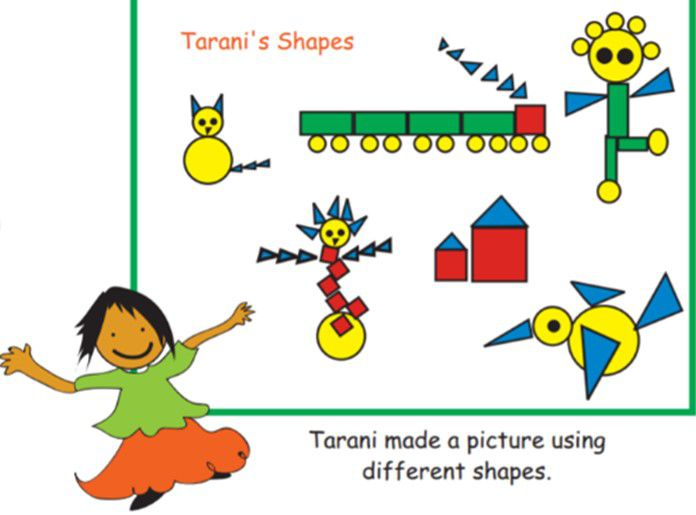Look at the above picture and answer the following: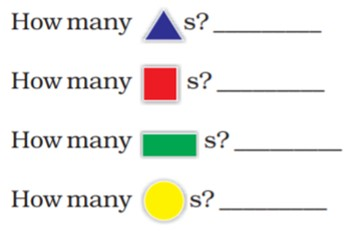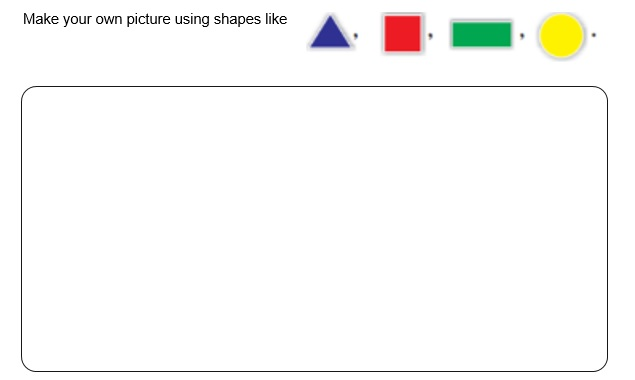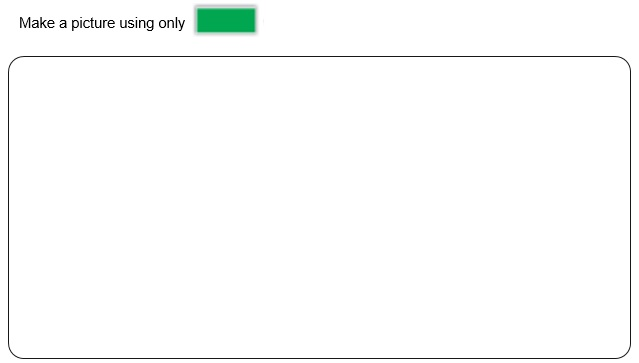# Practice these questions

Q1) Count these shapes: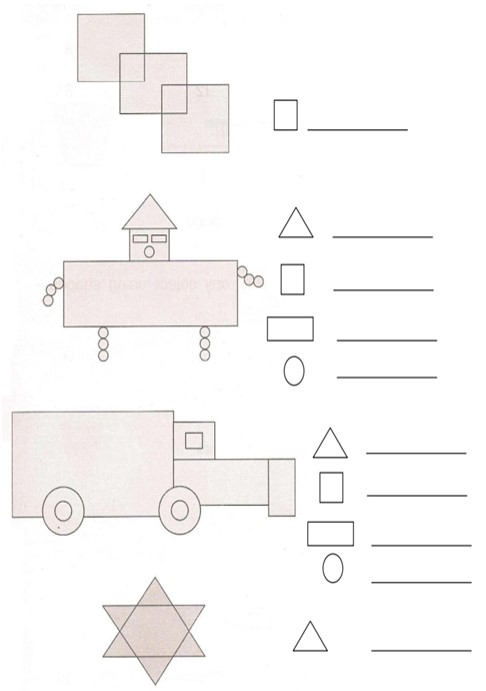Q2) Write the names of the things you can find with these shapes.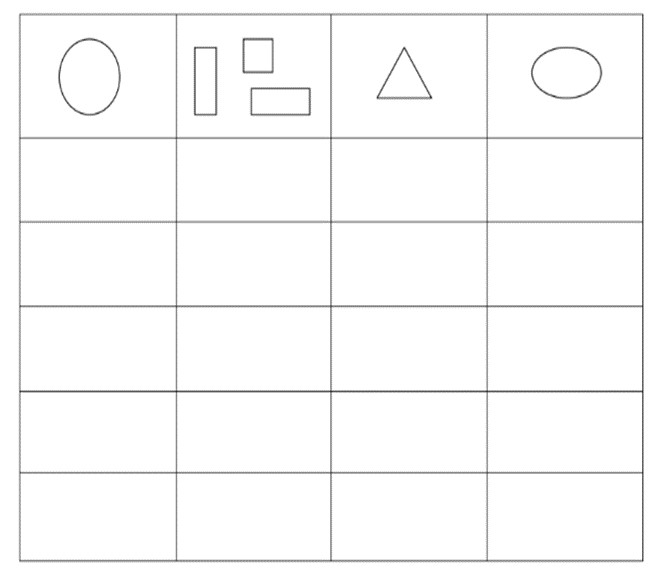## Quiz for Point, Line and Plane Figures

 Q.1 A circle has _____ sides. a) 0 b) 2 c) 3 d) 4
 Q.2 Identify the figure.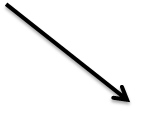a) Ray b) Line c) Line Segment d) Point
 Q.3 Identify the figure?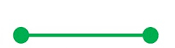a) Ray b) Line c) Line Segment d) Point
 Q.4 Identify the figure?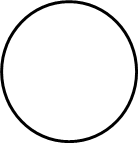a) Circle b) Rectangle c) Triangle d) Square
 Q.5 Identify the figure?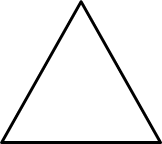a) Circle b) Rectangle c) Triangle d) Square
 Q.6 A quadrilateral with 5 sides is known as _______. a) Circle b) Pentagon c) Square d) Hexagon
 Q.7 Identify the figure?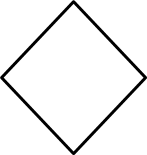a) Diamond b) Square c) Circle d) Triangle
 Q.8 Identify the figure?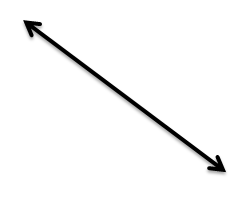a) Line b) Line Segment c) Ray d) Point
 Q.9 In a rectangle, the pair of opposite sides are _________________. a) Unequal b) Intersecting c) Equal d) Similar
 Q.10 How many vertices does this plane figure have?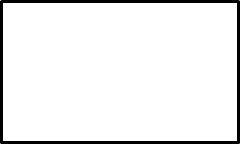a) 4 b) 3 c) 6 d) 5